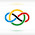### 2020 ISL #A2

Let $\mathcal{A}$ denote the set of all polynomials in three variables $x, y, z$ with integer coefficients. Let $\mathcal{B}$ denote the subset of $\mathcal{A}$ formed by all polynomials which can be expressed as \begin{align*} (x + y + z)P(x, y, z) + (xy + yz + zx)Q(x, y, z) + xyzR(x, y, z) \end{align*}with $P, Q, R \in \mathcal{A}$. Find the smallest non-negative integer $n$ such that $x^i y^j z^k \in \mathcal{B}$ for all non-negative integers $i, j, k$ satisfying $i + j + k \geq n$.

The answer is $4$.

We first prove that $n \ge 4$. It suffices to show that $x^2y$ is not expressible. For the sake of contradiction, suppose it was. Plugging in $z=-x-y$ gives $\big(xy-(x+y)^2\big)Q(x,y,-x-y) + xy(-x-y)P(x,y,-x-y)=x^2y.$Since the RHS has degree $3$, we know that all non-degree $3$ terms on the LHS must be $0$. Solely considering the degree $3$ terms on the LHS, we have $(x^2+xy+y^2)(c_1x + c_2y) + xy(x+y)d_1 = x^2y$for some constants $c_1,c_2,d_1$. Expanding, we note that the coefficients of $x^2y$ and $xy^2$ are equal on the LHS, which is a contradiction. Thus, no $n\le 3$ work.

Now we show our construction for $n=4$. In other words, we must show that $n=4$ works. We will prove that any monomial of degree $n\ge 4$ is expressible. Let this monomial be $x^ay^bz^c$.

Case 1: $a,b,c\ge 1$. This is divisible by $xyz$, so just use $R$.

Case 2: $c=0$ and $a,b\ge 1$. (Other such cases follow by symmetry.)

Subcase 2.1: $a,b\ge 2$. Then$x^ay^b=x^{a-1}y^{b-1}(xy+yz+zx)-(x^{a-2}x^{b-1}-x^{a-1}y^{b-2})xyz.$

Subcase 2.2: $a=1$ and $b\ge 2$. (The case $b=1$ and $a\ge 2$ is analogous.) In fact, $b\ge 3$ since $xy^b$ must have degree at least $4$. Notice$xy^b=xy^{b-1}(x+y+z)-x^2y^{b-1} - xy^{b-1}z.$The second term is expressible from Subcase 2.1, and the last term is divisible by $xyz$, done.

Case 3: $c=0,b=0,a\ge 4$. (Other such cases follow by symmetry.) We want to construct $x^a$. Notice$x^a=(x+y+z)x^{a-1} - x^{a-1}y - x^{a-1}z.$The final two terms are expressible by Subcase 2.2, and we are done.

In all cases, $x^ay^bz^c$ is expressible, so we have proved that $n=4$ works.

We are done. $\square$

1.Anonymous3/21/2022

dude
my heart skips a beat when I see the notification of a new post

2.I'm really glad to know that you like my posts :)

3.Anonymous3/22/2022

@Anonymous

Mine skips two

4.Anonymous3/22/2022

y'all suck

mine skips pi beats

5.Anonymous3/29/2022

waiting for the next post :(

6.Anonymous7/04/2022

hi

1.hello!